#### You may also like### Fibs

The well known Fibonacci sequence is 1 ,1, 2, 3, 5, 8, 13, 21.... How many Fibonacci sequences can you find containing the number 196 as one of the terms?### Paving Paths

How many different ways can I lay 10 paving slabs, each 2 foot by 1 foot, to make a path 2 foot wide and 10 foot long from my back door into my garden, without cutting any of the paving slabs?### 1 Step 2 Step

Liam's house has a staircase with 12 steps. He can go down the steps one at a time or two at time. In how many different ways can Liam go down the 12 steps?

# Fibonacci Surprises

##### Age 11 to 14Challenge Level
Lots of people submitted solutions to this problem - thank you everyone! There were too many good solutions to name everybody, but we've used a selection of them below:

Most of you decided to start with some examples. This is how Tess, Tilli and Clem, from the International Grammar School, Australia, Ryan and Kian, from Oundle and King's Cliffe Middle School, and Ruby, from St Philip's Primary School, started this problem:

1. Adding three consecutive Fibonacci numbers: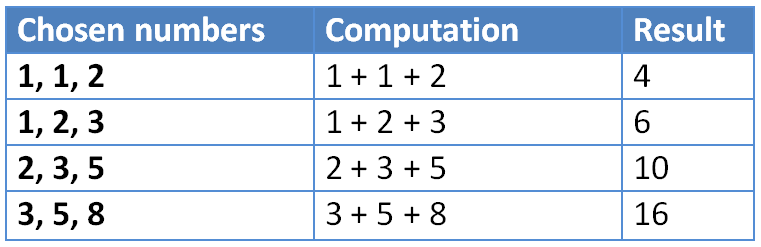2. Adding the first and last from four consecutive Fibonacci numbers, then dividing by two: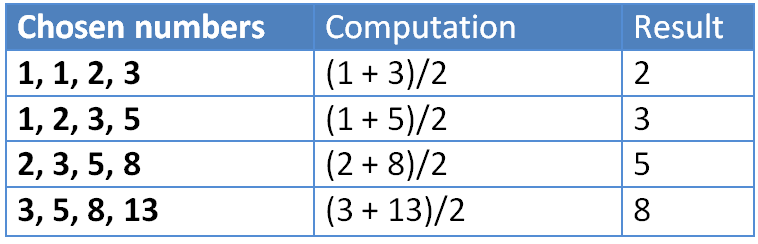3. Adding six consecutive Fibonacci numbers and dividing by four: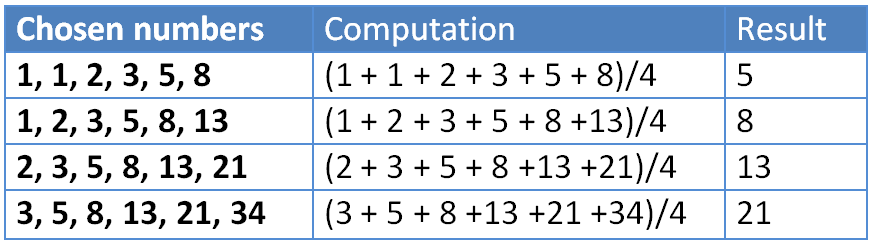Brian and Hugh, from DCB, South Korea, and Irie-Rose, from St Phillip's Primary School, made some observations about the pattern of odd and even numbers:

We noticed that the numbers in the Fibonacci sequence appear in a pattern regarding odd and even numbers. The Fibonacci sequence is: ODD, ODD, EVEN, ODD, ODD, EVEN, ...

This made sense, since it starts with two odd numbers ($1, 1$) and the sum ODD + ODD = EVEN and EVEN + ODD = ODD.

1. For the first sequence, we noticed that all the resulting numbers are even. This makes sense since, from any three consecutive Fibonacci numbers, one is even and the other two are odd (which follows from our initial observation). Therefore, their sum will be an even number.

2. The second sequence is a Fibonacci sequence starting from $2$ and $3$, so the pattern of odd and even numbers is: EVEN, ODD, ODD, EVEN, ODD, ODD, ...

Also, all the numbers are integers, since, from our initial observation, if we choose four consecutive Fibonacci numbers, then the first and the last will either be both odd or both even, so their sum will be even.

3. This time the pattern is a Fibonacci sequence starting from $5$ and $8$, so the pattern is: ODD, EVEN, ODD, ODD, EVEN, ODD, ...

Mikaeel, from Marlborough Primary School, Lera and Ahmed, from Harbinger School, and Ashley, from Brookfield Community School, noticed that the numbers are in a Fibonacci sequence:

1. The first sequence of numbers is formed as a Fibonacci sequence, but starts from $4$ and $6$ instead of $1$ and $1$.

2. This gives the usual Fibonacci sequence, but without the first two terms.

3. This gives the usual Fibonacci sequence, but without the first four terms.

Spiros and George, from Hazelwood School, and Yash, from Tanglin Trust School, Singapore explained why we end up with a Fibonacci sequence:

From here on, $F_n$ will be used to denote the $n^{\text{th}}$ term of the usual Fibonacci sequence.

1. Three consecutive terms of the new sequence would be: $F_n + F_{n+1} + F_{n+2}$, $F_{n+1} + F_{n+2} + F_{n+3}$ and $F_{n+2} + F_{n+3} + F_{n+4}$. But the first term of our third number, is the sum of the first terms of our first two numbers (i.e. $F_{n+2} = F_n + F_{n+1}$). Likewise, the second term of the third number is the sum of the second term of the previous two numbers, and similarly for the third number.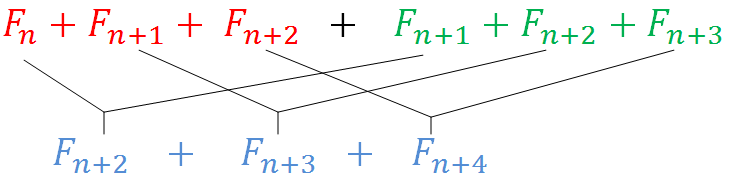Therefore, each number from our sequence is the sum of the two previous numbers. Also it starts from $4$ and $6$. So it is a Fibonacci sequence starting from $4$ and $6$.

2. We use the same idea as before. This time our three consecutive numbers in the sequence are: $\frac{F_n+F_{n+3}}{2}$, $\frac{F_{n+1}+F_{n+4}}{2}$ and $\frac{F_{n+2}+F_{n+5}}{2}$. We can still conveniently add the terms in the sum of the first two numbers in order to get the third, as shown in the diagram below: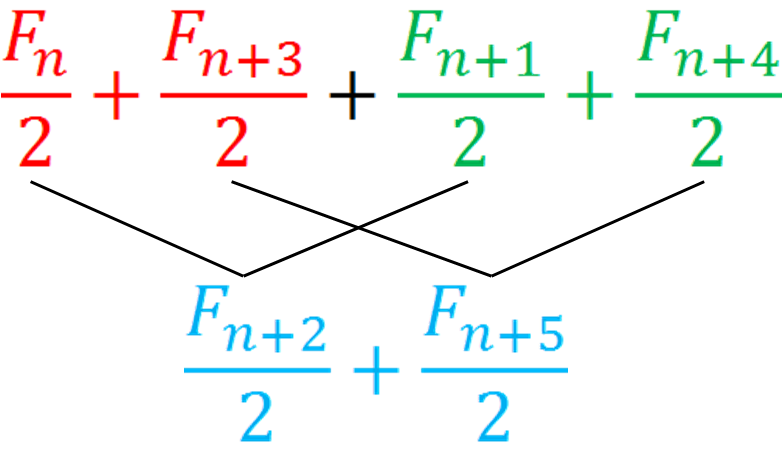Hence, each number from our sequence is the sum of the two previous numbers. Also, it starts from $2$ and $3$ so is the usual Fibonacci sequence without the first two terms.

3. We do something similar for the last one as well: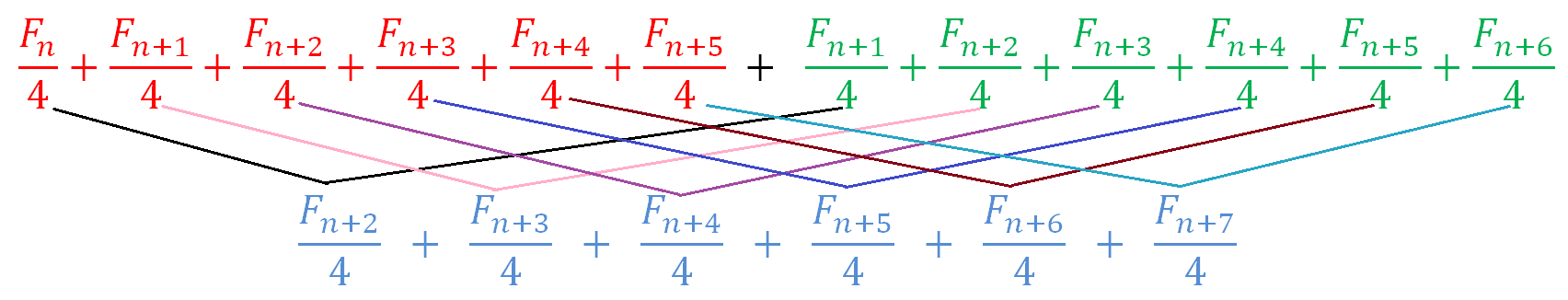So we can obtain the third number in our sequence by adding the prevoius two. Hence, it is a Fibonacci sequence starting from $5$ and $8$, i.e. a Fibonacci sequence without the first four terms.

Amy, Emily, Rachael, Hollie, Daisy, Eleanor, Holly, Henry, Charlie and Elliot from Oundle and King's Cliffe Middle School, Nina, Hannah and Bronwen from St Philip's Primary School and Matthew and Benjamin from Tanglin Trust School, Singapore observed some rules in terms of the Fibonacci terms used:

1. When adding up three consecutive Fibonacci terms, we end up with twice the third number.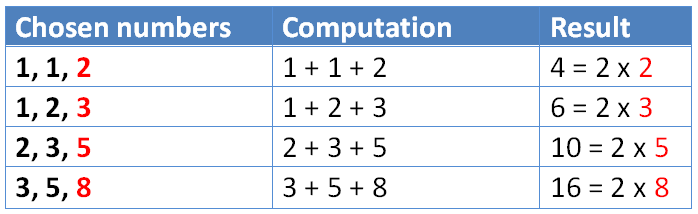2. When choosing four consecutive Fibonacci numbers, adding the first and the last and dividing by $2$, we end up with the penultimate number.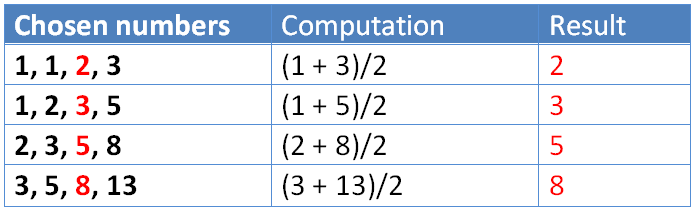3. When adding up six consecutive Fibonacci numbers and dividing by $4$ we and up with the fifth.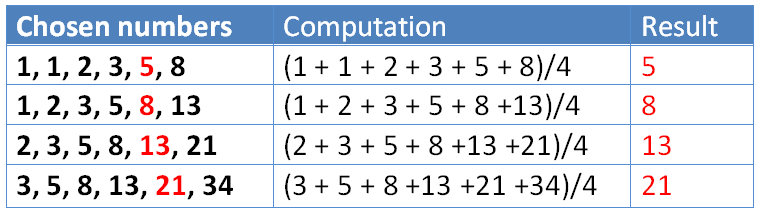Farden and Len, from Drayton Manor High School, Abi, Poppy and Olivia from Ousedale School and Zach explained why this happens:

1. We want to choose, three consecutive Fibonacci numbers. If the first two are $a$ and $b$, the third one will be $a+b$, since every number is the sum of the previous two. Therefore their sum will be $a + b + (a+b) = 2(a+b)$, so their sum equals doulbe the last of the Fibonacci numbers chosen.

2. We now have to choose four terms. If the first two are $a$ and $b$, the third will be $a+b$ and the fourth will be $b+(a+b) = a +2b$. Now, if we add the first and the last and divide by 2, we get: $\frac{a + (a+2b)}{2} = \frac{2(a+b)}{2} = a+b$. So the result is the third number.

3. We do something similar for the last sequence. We need to choose six consecutive Fibonacci numbers. We again let the first two be $a$ and $b$. The next ones will then be $a+b$, $a+2b$, $(a+b) +(a+2b) = 2a+3b$, and $(a+2b) + (2a+3b) = 3a + 5b$. We now add all of them, to get: $a +b+(a+b)+(a+2b)+(2a+3b)+(3a+5b) = 8a +12b$. If we divide the result by $4$ we get $\frac{8a+12b}{4} =2a+3b$, which is the penultimate number.

Nia, from School No 97, Bucharest, Romania, proved it in a different way:

1. We want to add three consecutive Fibonacci numbers, say $F_n, F_{n+1}$ and $F_{n+2}$. Their sum will be $(F_n +F_{n+1}) + F_{n+2} = F_{n+2} +F_{n+2} = 2 \times F_{n+2}$, since $F_n +F_{n+1} = F_{n+2}$.

2. We choose four consecutive Fibonacci numbers, say $F_n, F_{n+1}, F_{n+2}$ and $F_{n+3}$. We now add the first and the last together and we get $F_n +F_{n+3} = F_n +F_{n+1}+F_{n+2}$, since $F_{n+3} = F_{n+1}+F_{n+2}$. So the sum of the first and the fourth is the same as the sum of the first three numbers. So this is the same as the sum in the first sequence, so it is equal to $2 \times F_{n+2}$. Therefore, if we divide by $2$ the result will be $F_{n+2}$.

3. Six consecutive Fibonacci numbers can be written as: $F_n, F_{n+1}, F_{n+2}, F_{n+3}, F_{n+4}$ and $F_{n+5}$. Their sum will then be $F_n+ F_{n+1}+ F_{n+2}+ F_{n+3}+ F_{n+4}+F_{n+5}$. We replace $F_n + F_{n+1}$ with $F_{n+2}$, and $F_{n+5}$ with $F_{n+4} + F_{n+3}$ and the sum becomes: $2F_{n+2} + 2F_{n+3}+ 2F_{n+4}= 2(F_{n+2} + F_{n+3} + F_{n+4})$.

Hence, the result is twice the sum of three consecutive Fibonacci numbers. By the observations for the first sequence, the sum of three consecutive Fibonacci numbers is twice the last one. Therefore, $F_{n+2} + F_{n+3} + F_{n+4} = 2 F_{n+4}$, so the sum of the six consecutive Fibonacci numbers is $4F_{n+4}$. Now we divide the sum by $4$ and the result is $F_{n+4}$, i.e. the penultimate Fibonacci number.

Zach found some other Fibonacci Surprises. Can you use some of the methods above to explain why they happen?

1. The sum of any four consecutive Fibonacci numbers equals the sum of the third and the fifth.

2. Take any set of $5$ consecutive Fibonacci numbers, sum the first and fifth numbers, divide by $3$ to find the third number in that set.

3. Take any set of $7$ consecutive Fibonacci numbers, sum the first and seventh numbers, divide by $2$ to find the sum of the third and fifth number in that set.

4. Sum any set of $8$ consecutive Fibonacci numbers, divide by $3$ to find the sum of the fifth and seventh number in that set.

5. Take any set of $7$ consecutive Fibonacci numbers, subtract the first from the last number, divide by $4$ to find fourth number in that set.

6. Take any set of $9$ consecutive Fibonacci numbers, subtract the first from the ninth number, divide by $3$ to find the sum of the fourth and sixth number in that set.

7. Take any set of $9$ consecutive Fibonacci numbers, add the first to the ninth number, divide by $7$ to find the fifth number in that set.

8. Sum any set of $10$ consecutive Fibonacci numbers, divide by $11$ to find the seventh number in that set.

9. Take any set of $10$ consecutive Fibonacci numbers, add the first to the tenth number, divide by $2$ to find the sum of the third, fifth and eighth numbers in that set.

Thank you again and well done to everybody who submitted a solution!